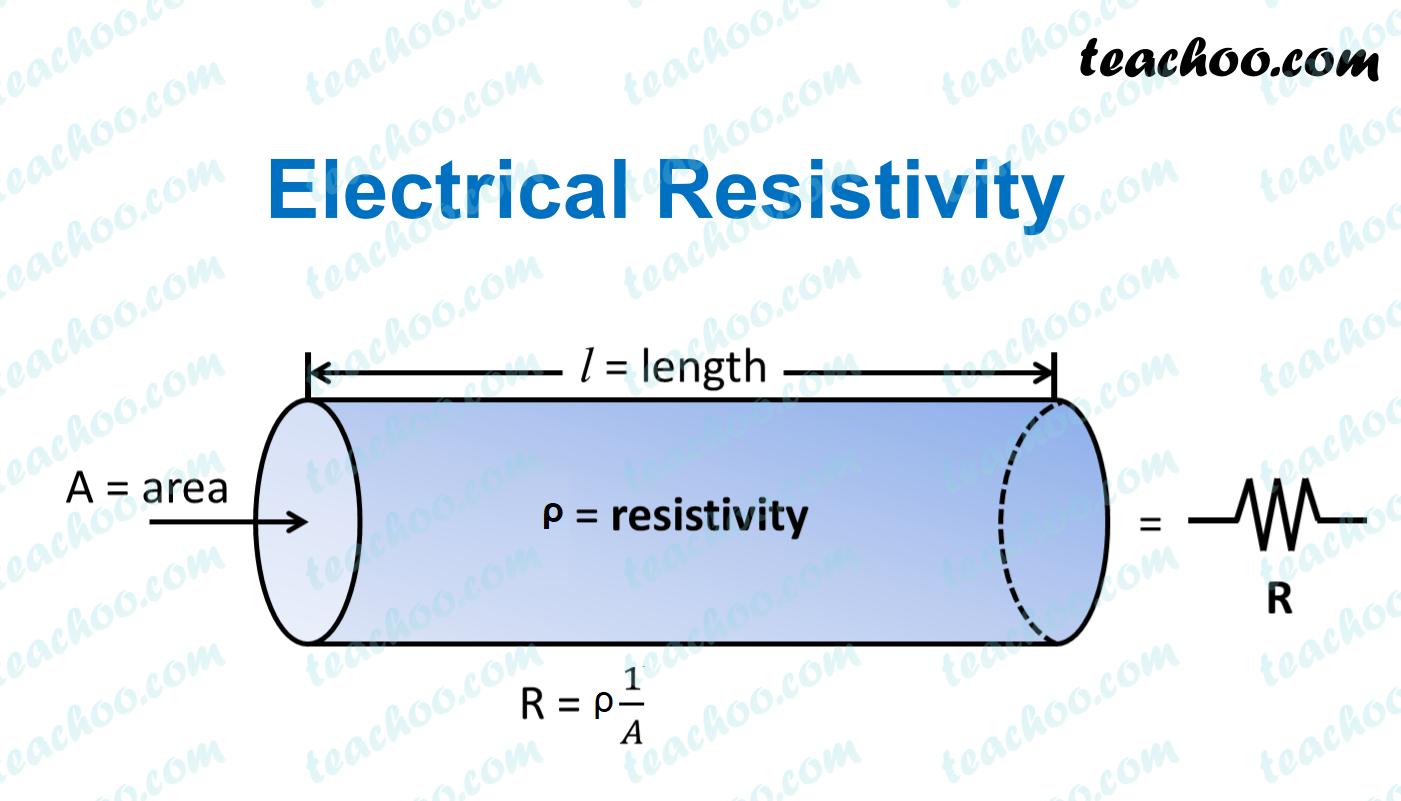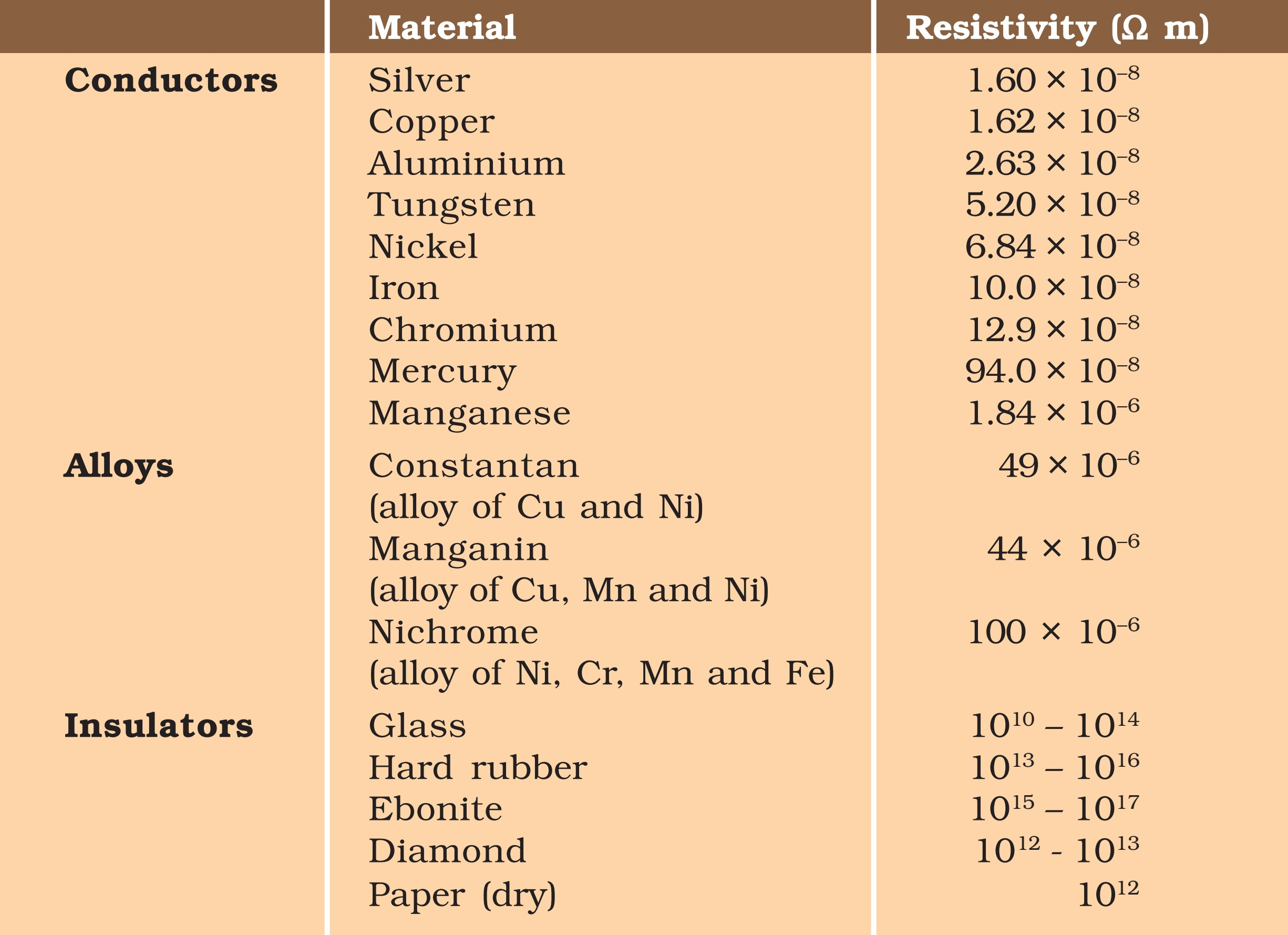Concepts

Class 10
Chapter 12 Class 10 - ElectricityResistivity is the characteristic property of the material by which it resists the amount of current through it.

## What is the SI Unit of resistivity?

SI Unit of resistivity is ohm meter.

Explanation.

We know that,

R = ρ (l/A)

Therefore,

ρ = R (A/l)

= ohm × m 2 /m

= ohm × m

Therefore, unit of rho is ohm meter.

Note: Unit of R is ohm, Unit of area of cross-section is m 2 , unit of length is m

## Factors on which resistivity depends

Resistivity is a characteristic property of the material.

It depends upon

• Nature of material
• Physical conditions, like temperature

On the basis of resistivity of material, different materials can be categorized into

• Conductors - Those materials which have very low resistivity and allow the current to pass through them easily. Eg- copper.
• Insulators - Those materials which have very high resistivity and do not allow the current to pass through them easily. Eg-plastic.

There are some materials whose resistivity lies between that of conductors and insulators.

They are called alloys.

Alloys are made up with one or more than one metals. (Eg Nichrome)

Alloys have various properties dues to which they are used as heating elements in various appliances .Note - Resistivity of an Item does not depend upon length of wire or Area of Cross Section
It remains constant for a particular material.
(It is the Resistance which changes with length of wire/Area of Cross Section)

Learn in your speed, with individual attention - Teachoo Maths 1-on-1 Class